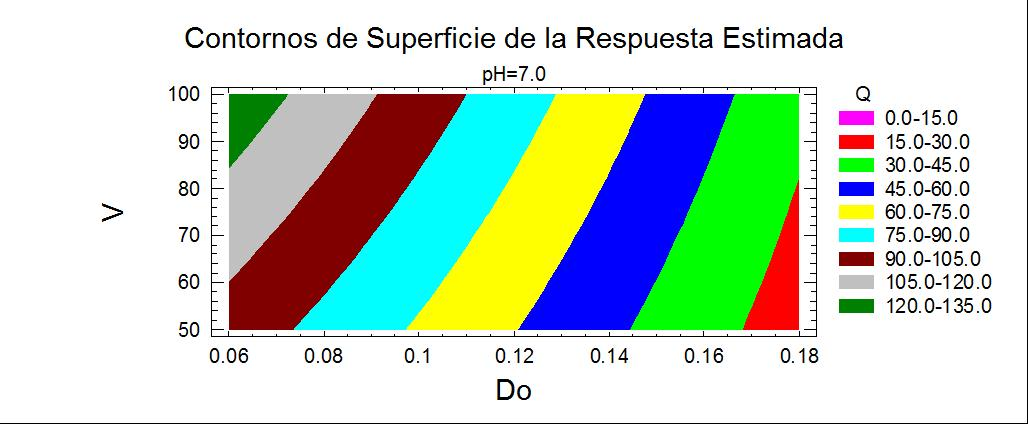BIOSORCION DE PLOMO DE AGUAS CONTAMINADAS UTILIZANDO Pennisetum clandestinum Hochst (KIKUYO)(LEAD BIOSORTION OF CONTAMINATED WATERS USING Pennisetum clandestinum Hochst ( KIKUYU))

Amanda Maldonado, Celestino Luque, Duvalier Urquizo

Online: 29-04-2012Abstract

Se investigó la remoción de plomo de un agua simulada con 30 ppm Pb (II) utilizando el kikuyo como biosorbente preparado mediante un proceso de hidrólisis acida seguido de una hidrólisis básica. El proceso de adsorción, se realizó en un equipo de prueba de jarras, donde se estudiaron las variables independientes: dosis del biosorbente, velocidad de agitación y pH, y como variable respuesta la capacidad de adsorción (Q). Se logró una capacidad máxima de adsorción de 139.35 mg/g con 0.06 g de dosis de biosorbente (kikuyo), 100 rpm para velocidad de agitación y pH 6. Se determinó el modelo matemático que relaciona Q, con la variable más significativa, dosis del biosorbente (Do). La cinética de adsorción, obedece a un modelo de primer orden (R2 = 0.9445); donde el valor de k = 0.0089 t-1. El modelo de Langmuir es el que representa el proceso de adsorción ( R2= 0.9955).

The removal of lead from a 30 ppm Pb (II) simulated water using Kikuyu as a biosorbents that was prepared by a process of acid hydrolysis followed by a basic hydrolysis was investigated. The adsorption process was conducted in a jar test equipment in order to find out the relationships between the adsorption capacity (Q), the response variable, and the independent variables under study: biosorbents dose, stirring speed and pH. It was found that the maximum capacity of adsorption of 139.35 mg / g was achieved with 0.06 g of biosorbents dose (Kikuyu), 100 rpm for stirring speed and pH 6. The mathematical model that relates Q with the most significant variable, the biosorbents dose (Do) was determined.The kinetics of adsorption follows a first order model (R2 = 0.9445), where the value of k = 0.0089 t-1.The Langmuir model represents the adsorption process ( R2 = 0.9955).